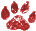# Subclass Depth Init Speed

 Namespace: WIN_COM_API
It's been asserted that the depth of class hierarchies alone has a negative impact on instantiation time. This is not supposed to be the case in VFP 6. The code below demonstrates this. Note that each subclass contains custom code as well as a custom property setting and that the custom code would force program execution to drill all the way up the class hierarchy.
I recall doing benchmarks in VFP 5 and VFP 3 and if memory serves me well, subclass speed has always been almost independent of depth alone.-- Steven Black
I changed the code to use a form class, since what we were discussing is Form Load Time. I also added code to include a 500 line procedure in each class definition, which causes over 25,000 lines of code to be generated. The impact on instantiation time? Zero! The presences of code and the depth of class hierarchies has little or no impact on instantiation time, in fact in this example, instantiating a class 25 levels deep in the hierarchy takes the same amount of time as 3 levels deep. Again, the mere presence of code and the depth of the class hierarchy have little or no impact, it's the amount of code run (and the efficiency of that code) that matters. -- Mike Feltman

```CLEAR ALL
CLOSE ALL
CLEAR
#DEFINE NUMCLASSES 25
#DEFINE CR CHR(13)

lcString = ""
FOR lnI = 1 TO NUMCLASSES

lcString = lcString + "DEFINE CLASS test" + TRANSFORM(lnI) +" AS " + IIF(lnI=1,"Form","Test" + TRANSFORM(lnI-1) ) + CR
lcString = lcString + "Foo" + TRANSFORM(lnI) + " = " + TRANSFORM(lnI) + CR
lcString = lcString + "Function Init" + CR
lcString = lcString + "DODEFAULT()" + CR
lcString = lcString + "This.Foo" + TRANSFORM(lnI) + " = " + TRANSFORM(lnI) + CR
lcString = lcString + "ENDFUNC" + CR

lcString = lcString + "FUNCTION Dummy" + CR
FOR lnJ = 1 TO 500
lcString = lcString + "x = x + 1" + CR
NEXT
lcString = lcString + CR
lcString = lcString + "ENDDEFINE" + CR +CR
NEXT

STRTOFILE(lcString,"InitTest.prg")

COMPILE INITTEST
SET PROC TO InitTest

FOR lnI = 1 TO NUMCLASSES
lnStart = SECONDS()
loTest = CreateObject("Test" + TRANSFORM(lnI))
loTest.Show()
loTest.Release()
lnStop = SECONDS()
? "Depth " + TRANSFORM(lnI), "Init Time: " + STR(lnStop - lnStart,10,4)
NEXT

SET PROC TO
```

Contributors: Mike Feltman Steven Black
Category Performance
( Topic last updated: 2000.08.18 10:49:13 PM )